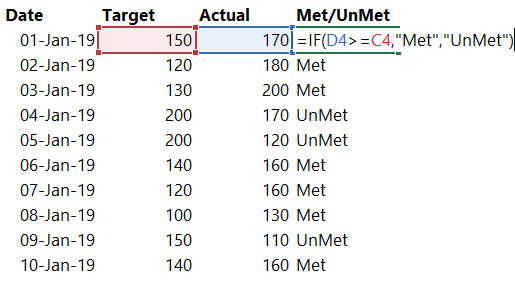The foremost function of Logic, IF, is pretty much a cake walk in Excel but this isn’t as intuitive as Power BI as in Excel. In this tutorial I’ll take you through various nuances of “correctly” using IF in DAX

### A quick glance at using IF in Excel…Note a few things about IF in Excel

1. The syntax of IF is almost the same in Excel as in DAX.
• In the condition Actual (cell D4) >= Target (cell C4) both the values are single/scalar (i.e. not a range or a table) values
• Also the words “Met” (Result if True) and “UnMet” (Result if False) is again a single/ scalar value
2. Since we write IF in each row of Excel we forget these obvious things
Circling back to IF in DAX, you can write IF in 3 different ways in DAX
1. In a column for each row of a table
2. In a measure
3. In a measure referring to each row of a table
I am sure you understood the excel part but in-case writing IF in DAX is turning into a nightmare for you. I’ll explain all the above 3 points in detail

### Writing IF in a column for each row of a Table

Consider the same table and writing an IF formulaWriting if in a column for each row of a table is exactly like Excel. Since IF comes in each row of the table this shouldn’t be a problem at all.

### Using IF in a Measure

This is where it begins to get tricky. Consider this (erroneous & incomplete) measureYou can see that I am half way through writing the measure but IF is not providing any intellisense dropdown to select the column of the table. This might have happened with you, the problem is..
• When you write a measure and try to select the column of the table, Power BI doesn’t know that which row are you referring to?
• Secondly your measure (if accepted) will yield a result with all the rows of the Data Table, but a measure can only have a single (scalar) value

How to get around this problem. 2 ways!
Method #1 (Writing IF in a Measure) – Use an aggregation to summarize the data, something like this
```Met/UnMet Measure Correct =
IF ( SUM ( Data[Actual] ) >= SUM ( Data[Target] ), "Met", "UnMet" )```

Note that..

• The SUM function converts the actual and target columns into a scalar value
• This measure is the not the same as writing if in each row of the table
Method #2 (Writing IF in a Measure) – To use the IF function in row context by using a row iterator like SUMX or FILTER
```Total of TagetMet Rows =
CALCULATE (
SUM ( Data[Actual] ),
FILTER ( Data, Data[Actual] >= Data[Target] )
)```
Another way of doing the same thing
```Total of TagetMet Rows =
SUMX ( Data, IF ( Data[Actual] >= Data[Target], Data[Actual] ) )```
In DAX unless you are using a single scalar value in all the 3 inputs in IF statement i.e.(Condition, Result if True, Result if False), it won’t work!